## Usage Patterns

### Simulink Patterns for `If`, `elseif`, `else` Constructs

These patterns shall be used for `if`, `elseif`, `else` constructs.

 Function Simulink pattern `if`, `elseif`, `else` construct when the Switch block is used. ```if (If_Condition) { output_signal = If_Value; } else if (Else_If_Condition) { output_signal = Else_If_Value; } else { output_signal = Else_Value; } ```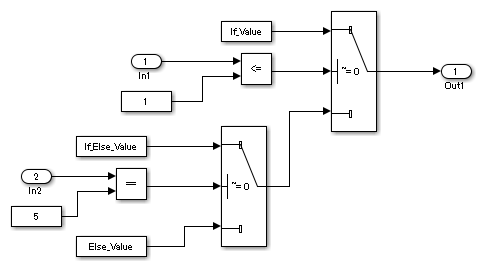`if`, `elseif`, `else` construct when using and action subsystem```if (Fault_1_Active & Fault_2_Active) { ErrMsg = SaftyCrit; } else if (Fault_1_Active | Fault_2_Active) { ErrMsg = DriverWarn; } else { ErrMsg = NoFaults; ```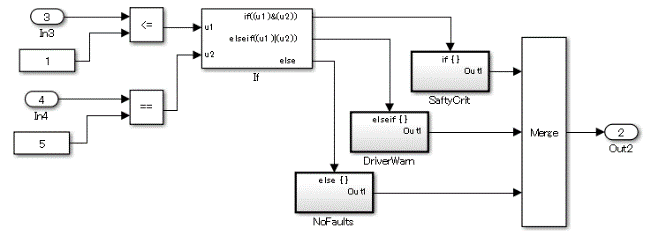### Simulink Patterns for `case` Constructs

These patterns shall be used for `case` constructs.

 Function Simulink Pattern Case construct using an action subsystem.```switch (PRNDL_Enum) { case 1 TqEstimate = ParkV; break; case 2 TqEstimate = RevV; break; default TqEstimate = NeutralV; break; } ```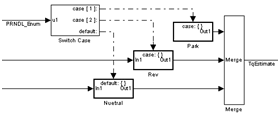Case construct using a Multiport Switch block.```switch (Selection) { case 1: output_signal = look1_binlxpw(In2,y1,x1,3U); break; case 2: output_signal = look1_binlxpw(In3,y2,x2,3U); break; case 3: output_signal = look1_binlxpw(In4,y3,x3,3U); break; default: output_signal = look1_binlxpw(In5,y4,x4,3U); break; } ```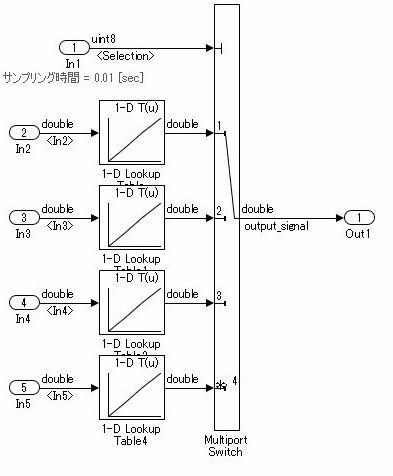### Simulink Patterns for Logical Constructs

These patterns shall be used for logical constructs.

Conjunctive normal formDisjunctive normal form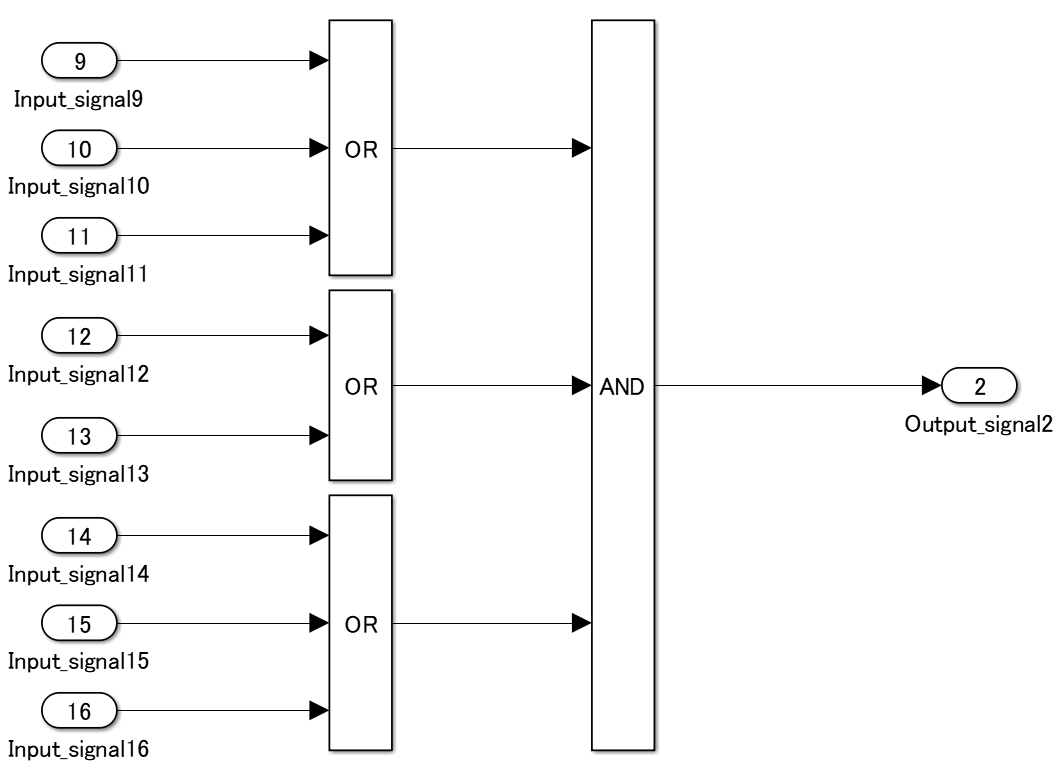### Simulink Patterns for Vector Signals

These patterns shall be used for vector signals.

 Function Simulink Pattern Vector signal and parameter (scalar) multiplication```for (i=0; i>input_vector_size; i++) { output_vector[i] = input_vector[i] * tunable_parameter_value; } ```(Reference: generated code of R2013b)```for (i = 0; i < input_vectorDim; i++) { output_vector[i] = tunable_parameter_value * input_vector[i]; } ``` (As the code is generated using a variable number of dimensions, the upper limit of the normal loop is a direct value.)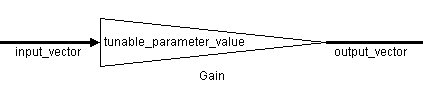Multiplication of vector signals and parameters (vectors) ```for (i=0; i>input_vector_size; i++) { output_vector[i] = input_vector[i] * tunable_parameter_vector[i]; } ```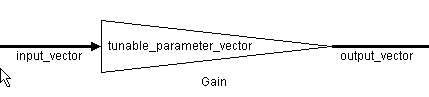Vector signal element multiplication```output_signal = 1; for (i=0; i>input_vector_size; i++) { output_signal = output_signal * input_vector[i]; } ```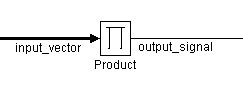Vector signal element division ```output_signal = 1; for (i=0; i>input_vector_size; i++) { output_signal = output_signal / input_vector[i]; } ```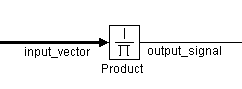Vector signal and parameter (scalar) addition ```for (i=0; i>input_vector_size; i++) { output_vector[i] = input_vector[i] + tunable_parameter_value; } ```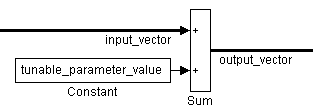Vector signal and parameter (vector) addition ```Vector signal and parameter (vector) addition for (i=0; i>input_vector_size; i++) { output_vector[i] = input_vector[i] + tunable_parameter_vector[i]; } ```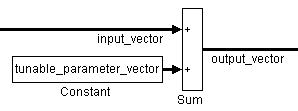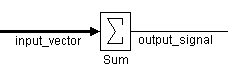Vector signal element subtraction ```output_signal = 0; for (i=0; i>input_vector_size; i++) { output_signal = output_signal - input_vector[i]; } ```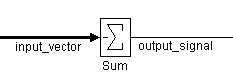Retention of minimum value/maximum value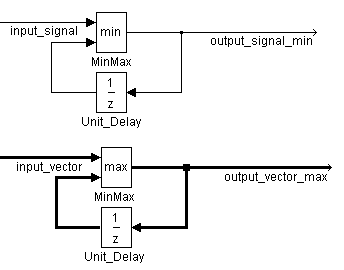### Using Switch and `If`, `Elseif`, `Else` Action Subsystems

The Switch block shall be used for modeling simple `if`, `elseif`,`else` structures when the associated `elseif` and `else` actions involve only the assignment of constant values.

Example — Recommended

For a simple `if`, `elseif`, `else` structure, use the Switch block.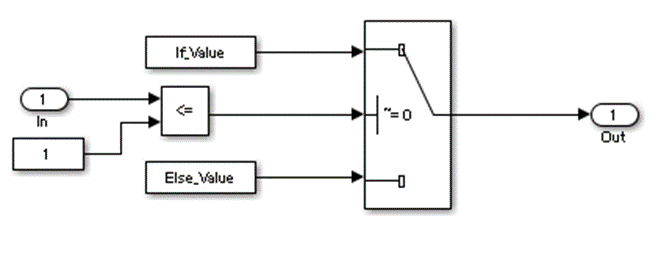Example — Not recommended

Using If and If Action Subsystem blocks for a simple `if`, `elseif`, `else` structure.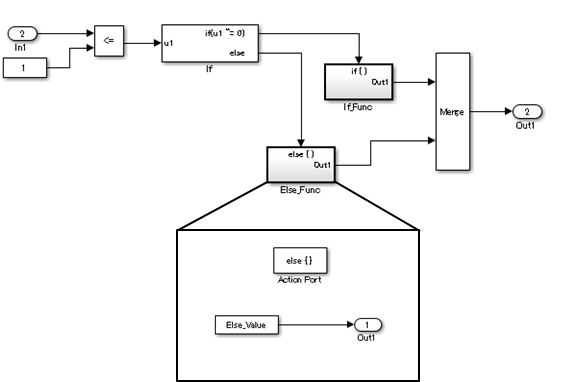Example — Recommended

For a complex `if`, `elseif`, `else` structure, use If and If Action Subsystem blocks.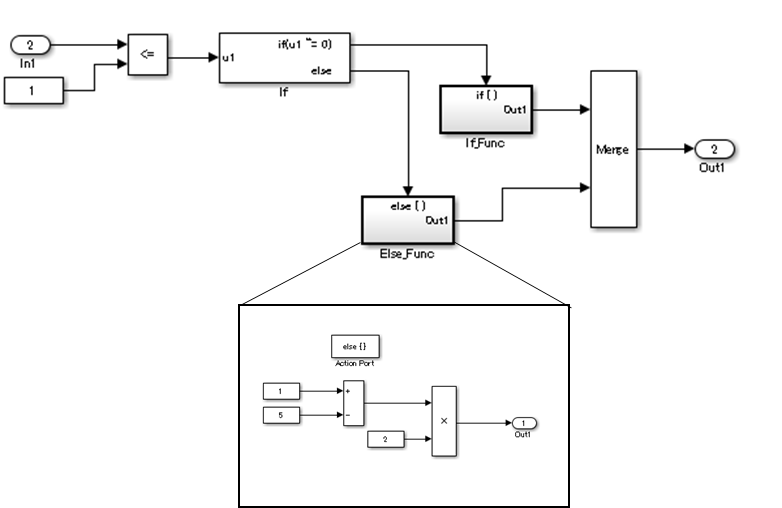Example — Not recommended

Using Switch block for a complex `if`, `elseif`, `else` structure.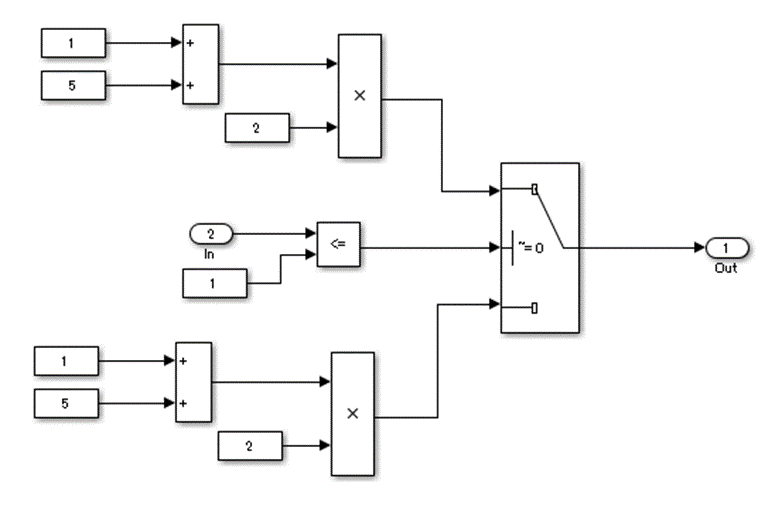### Use of `If`, `Elseif`, `Else` Action Subsystem to Replace Multiple Switches

Frequent use of the Switch block for condition bifurcation shall be avoided. Instead, the upper limit target shall be used (such as up to three levels). When the target value is exceeded, a conditional control flow using the `if`, `elseif`, `else` action subsystem shall be used.

Example — Not recommended

Four levels of nesting.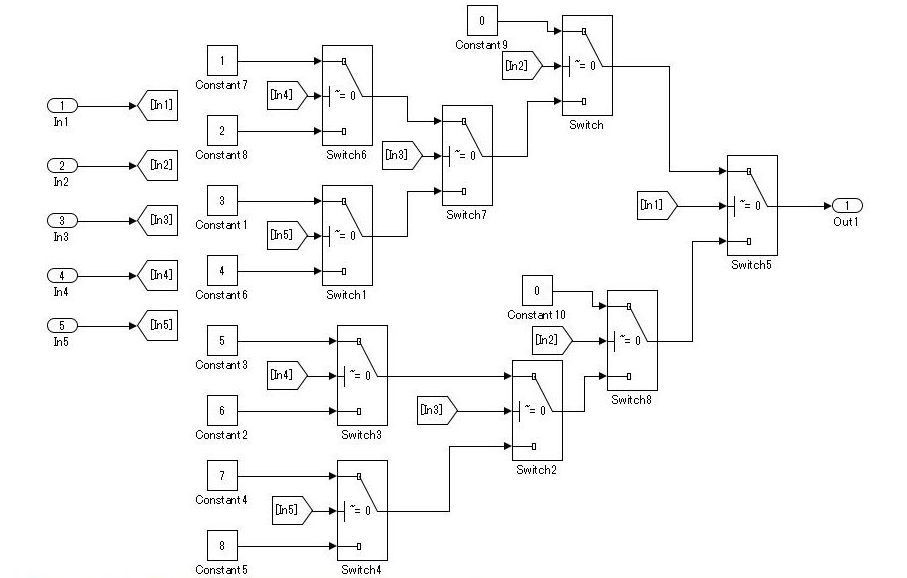Example — Recommended

By setting the fourth level as an `if` action subsystem, nesting is limited to a single level.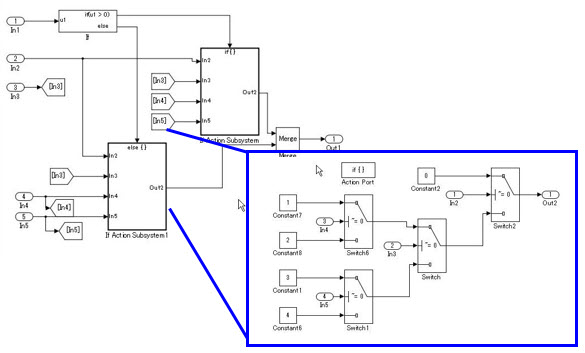Example — Not recommended

Not dividing by using an `if` action subsystem.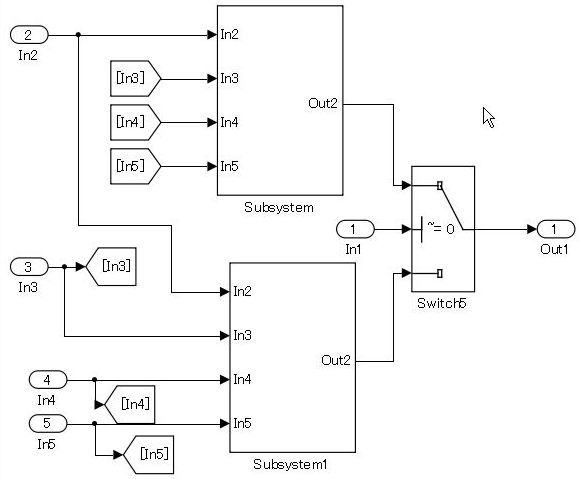Use atomic subsystem + function setting when the C code limit is applied. In this case, there is no need to use the `if`, `elseif`, `else` action subsystem, but the configuration of the Switch block can be split and encapsulated in the subsystem.

Example of model with five levels of nesting — Not recommended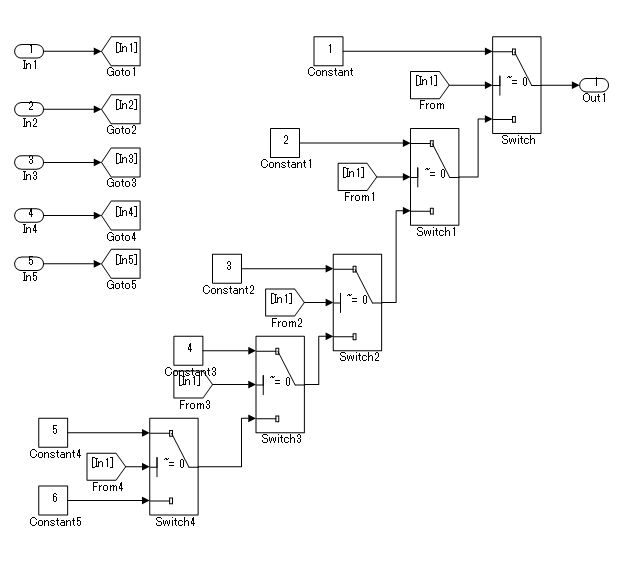Example of model with five levels of nesting — Recommended

Use a description method that avoids layering of nesting in the Switch block.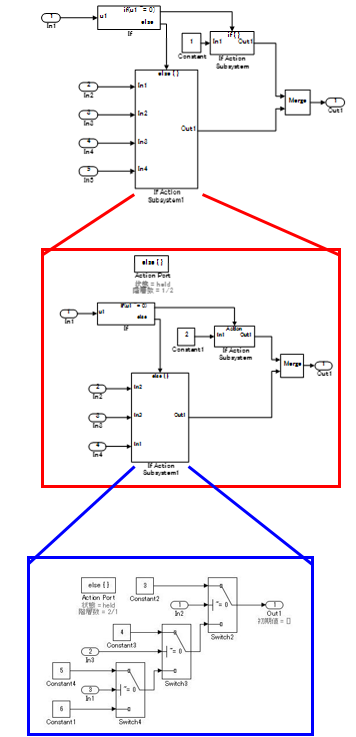While provided as an example, an `if` action subsystem is not typically used for switching the fixed value. In these Recommended and Not Recommended examples, the generated C code will be the same if the user does not add a function conversion setting. (Confirmed in R2010b to R2013a) The C code is unconstrained.

### Usage Rules for Action Subsystems Using Conditional Control Flow

Example — Recommended

An `if` action subsystem shall not be used when the associated actions do not have a status variable.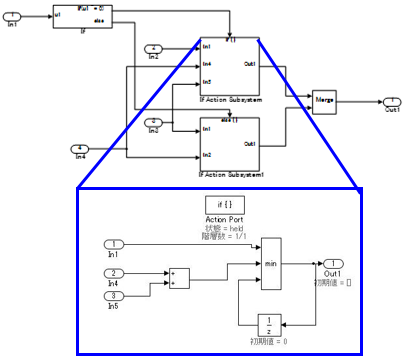Example — Recommended

Example of a model using five levels of nesting. Layering by using a subsystem does not occur because there is no internal state.Example — Recommended

An atomic subsystem is used to split either side of the Switch block without using an action subsystem.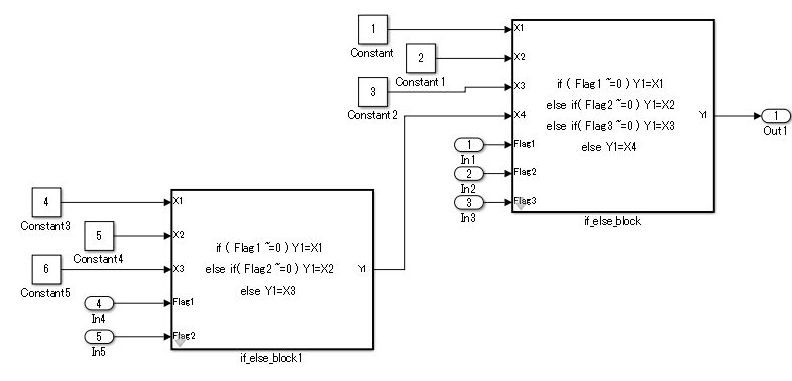Example — Not Recommended

Layering through the use of an unnecessary action subsystem.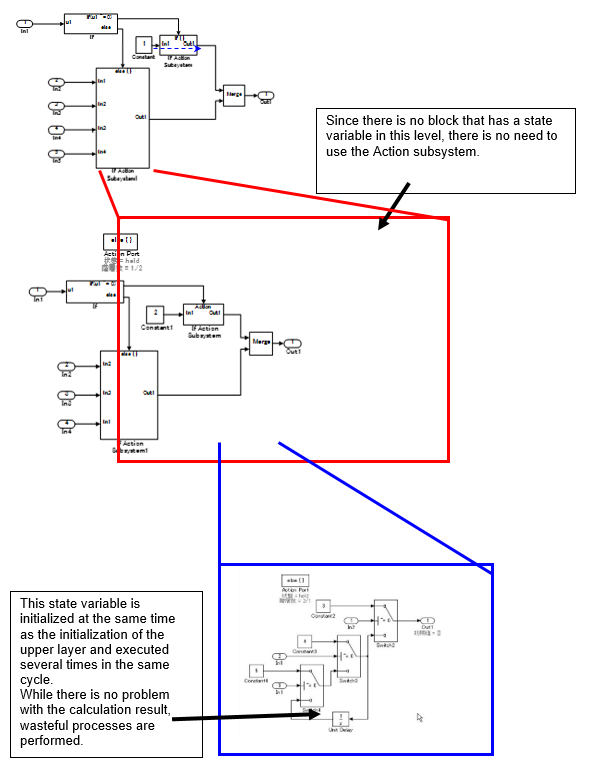If a function can be achieved by using the action subsystem, then layering using the action subsystem is not performed.

In the Not Recommended example, when the lowest level Unit Delay block on the third level is initialized, the conditional subsystem initialization is first executed one time on the upper first level, and then again on the second level for a total of two times of initial value settings. To prevent the generation of unnecessary code, it is recommended that listing not be made in conditional subsystems that reside in levels where the state variable does not exist.

This is based on the concept that the model complexity is reduced by dropping to a level. The purpose of the rule is to avoid the execution of unnecessary initializations.

For bifurcation of systems where the bifurcation condition nest has a deep structure, split by using function conversions to decrease the code bifurcation nesting. Functions before and after the Switch block are divided into respective subsystems, and function settings are applied to the atomic subsystem＋function. Be aware, it is possible that this may result in unintentional implementation and unnecessary RAM requirements.

### Test for Information From Errors

When functions that are used in Stateflow® (graphical functions, MATLAB® functions, etc.) results in an error, the error information shall be transformed into a model structure that will facilitate testing.

Not reviewing the error information returned by the functions can result in unintended behavior.

Example — Recommended

Error information is incorporated into the model structure, allowing the user to review and respond to the errors.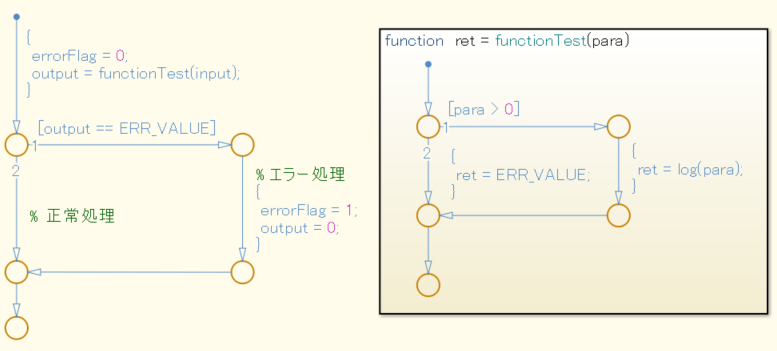Example — Not Recommended

Error information is not incorporated into the model structure.### Flow Chart Patterns for Conditions

These patterns shall be used for conditions within Stateflow flow charts.

 Function Flow Chart Pattern One condition. ```[condition] ```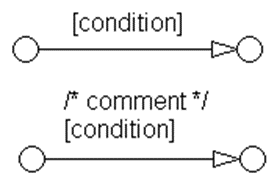Up to three conditions, short form.(The use of different logical operators in this form is not allowed. Use subconditions instead.)```[condition1 && condition2 && condition3] [condition1 || condition2 || condition3] ```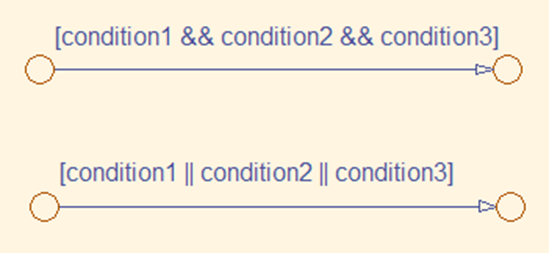Two or more conditions, multiline form. (The use of different logical operators in this form is not allowed. Use subconditions instead.) ```[condition1 ... && condition2 ... && condition3] [condition1 ... || condition2 ... || condition3] ```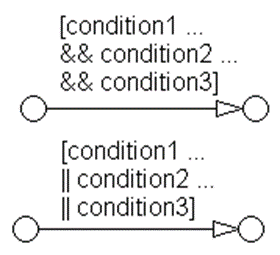Conditions with sub conditions. (The use of different logical operators to connect subconditions is not allowed. The use of brackets is mandatory.) ```[(condition1a || condition1b) ... && (condition2a || condition2b) ... && (condition3)] [(condition1a && condition1b) ... || (condition2a && condition2b) ... || (condition3)] ```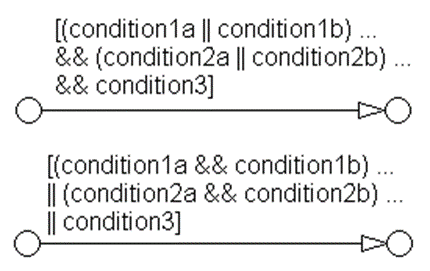Conditions that are visually separated. (This form can be combined with the preceding patterns.) ```[condition1 && condition2] [condition1 || condition2] ```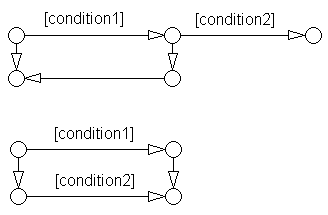### Flow Chart Patterns for Condition Actions

These patterns shall be used for condition actions within Stateflow flow charts

 Function Flow Chart Pattern One condition action.`action;`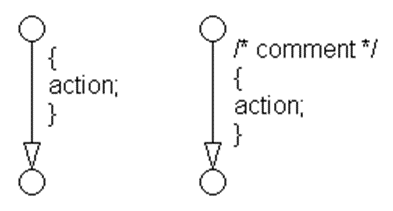Two or more condition actions, multiline form. (Two or more condition actions in one line are not allowed.) ```action1; ... action2; ... action3; ... ```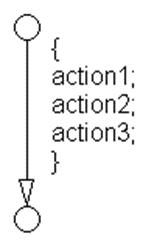Condition actions that are visually separated. (This form can be combined with the preceding patterns.) ```action1a; action1b; action2; action3; ```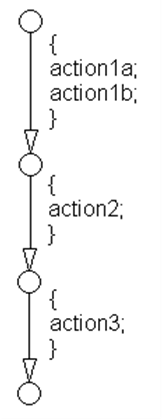### Flow Chart Patterns for `If`, `Elseif`, `Else` Constructs

These patterns shall be used for `If` constructs within Stateflow flow charts.

 Function Flow Chart Pattern `If` construct ```if (condition){ action; } ```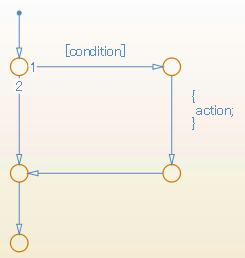`If`, `else` construct ```if (condition) { action1; } else { action2; } ```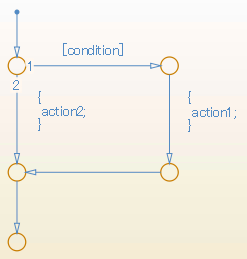`If`, `elseif`, `else` construct```if (condition1) { action1; } else if (condition2) { action2; } else if (condition3) { action3; } else { action4; } ```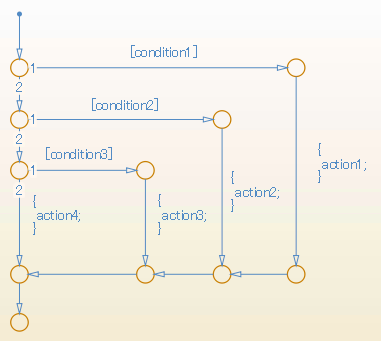Cascade of `if` construct.```if (condition1) { action1; if (condition2) { action2; if (condition3) { action3; } } } ```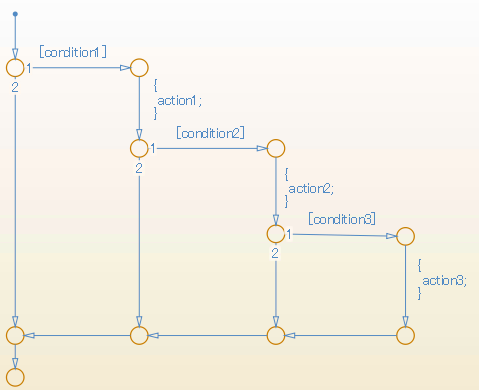### Flow Chart Patterns for Case Constructs

These patterns shall be used for case constructs in Stateflow flow charts.

 Function Flow Chart Pattern Case construct with exclusive selection. ```selection = u1; switch (selection) { case 1: y1 = 1; break; case 2: y1 = 2; break; case 3: y1 = 4; break; default: y1 = 8; } ```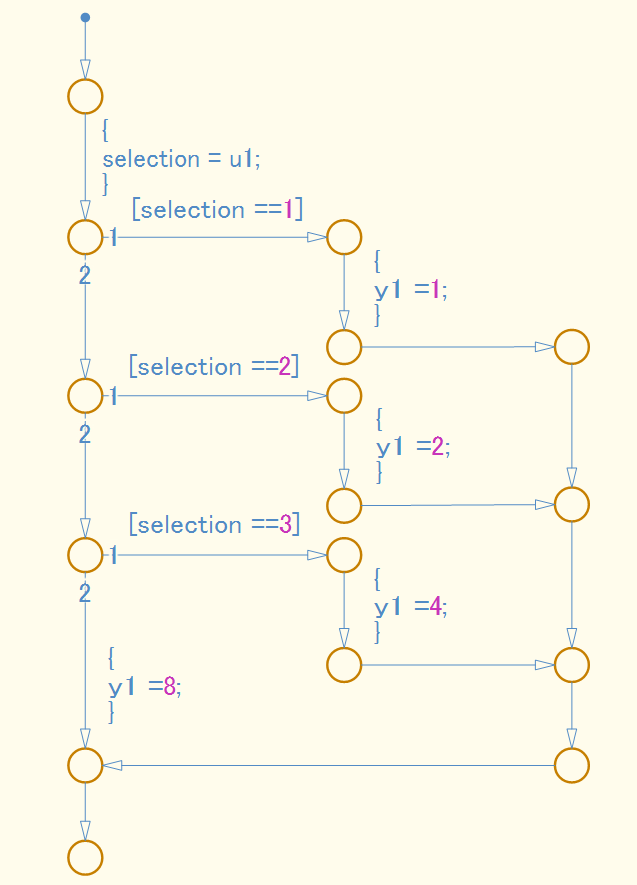Case construct with exclusive conditions. ```c1 = u1; c2 = u2; c3 = u3; if (c1 && ! c2 && ! c3) { y1 = 1; } elseif (! c1 && c2 && ! c3) { y1 = 2; } elseif (! c1 && ! c2 && c3) { y1 = 4; } else { y1 = 8; } ```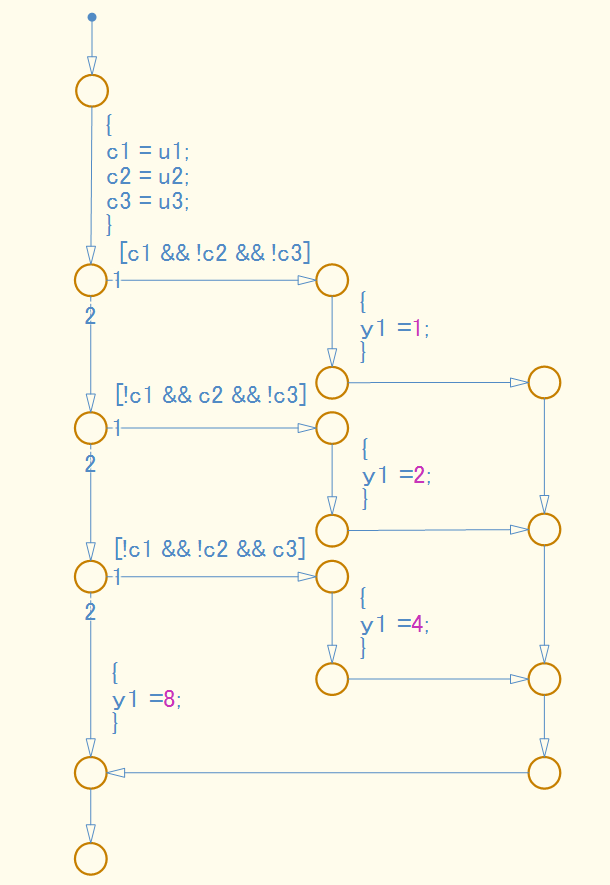### Flow Chart Patterns for Loop Constructs

These patterns shall be used to create loop constructs in Stateflow flow charts.

 Function Flow Chart Pattern For loop construct ```for ( index = 0; index < number_of_loops; index++ ) { action; } ```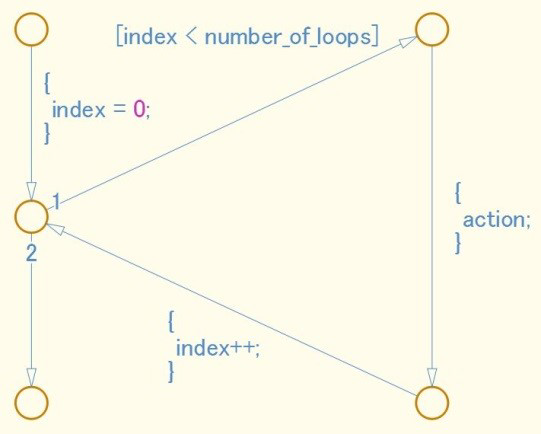While loop construct ```while ( condition ) { action; } ```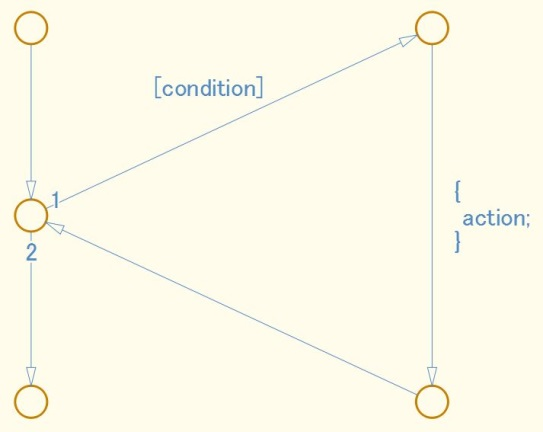Do While loop construct.```do { action; } while ( condition ) ```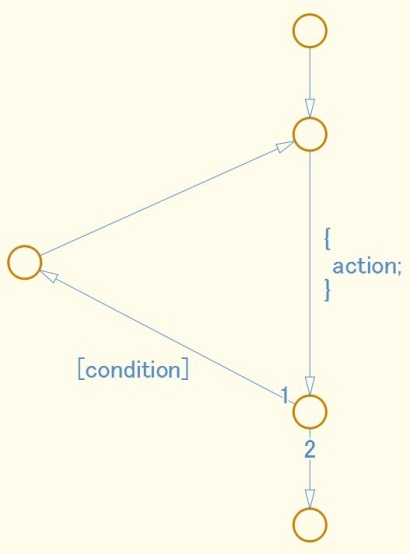### State Machine Patterns for Conditions

These patterns shall be used for conditions within Stateflow state machines

 Function State Machine Pattern One condition```(condition) ```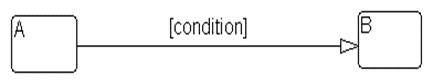Up to three conditions, short form(The use of different logical operators in this form is not allowed, use sub conditions instead) ```(condition1 && condition2) (condition1 || condition2) ```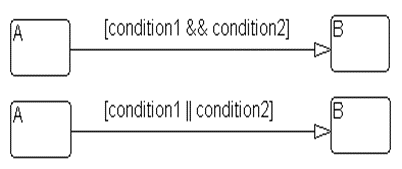Two or more conditions, multiline formA subcondition is a set of logical operations, all of the same type, enclosed in parentheses. (The use of different operators in this form is not allowed, use sub conditions instead.) ```(condition1 ... && condition2 ... && condition3) (condition1 ... || condition2 ... || condition3) ```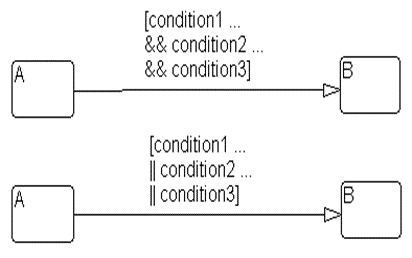### State Machine Patterns for Transition Actions

These patterns shall be used for transition actions within Stateflow state machines.

 Function State Machine Pattern One transition action. ```action; ```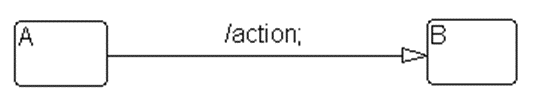Two or more transition actions, multiline form (Two or more transition actions in one line are not allowed.) ```action1; action2; action3; ```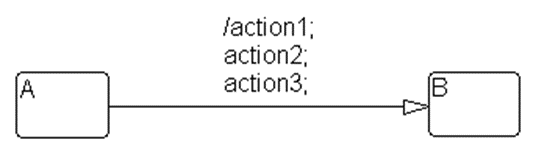### jc_0321Limiting State Layering

Within a single viewer (subviewer), multiple layering shall be limited by defining constraints for a single view (subview). Subcharts shall be used to switch the screen when defined constraint goals are exceeded.

Example — Recommended

The fourth level is encapsulated in a subchart.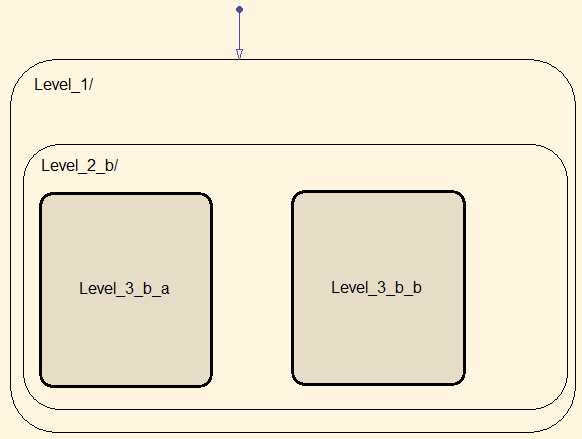Example — Not Recommended

The constraint goal is set to three levels, but Level_4_a and Level_4_b have more than three levels and are nested.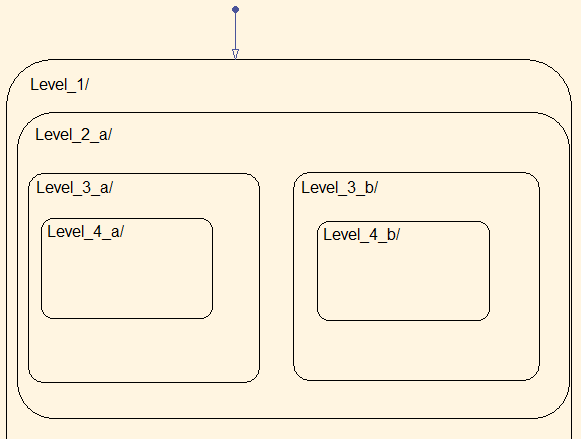### Number of States per Stateflow Container

The number of states per Stateflow container shall be determined by the number of states that can be viewed in the diagram. All states should be visible and readable.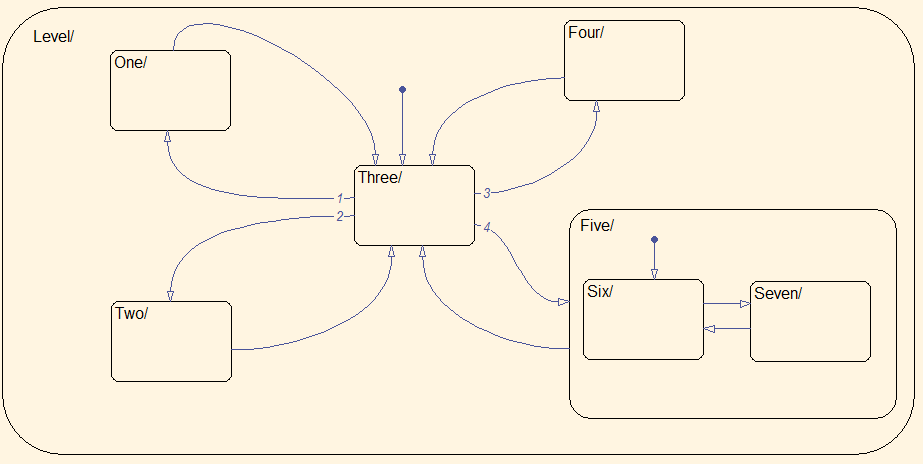### Function Call from Stateflow

If a state exists in the Function-Call Subsystem of the call target, and a “reset” of the state is required when the state of the caller becomes inactive, the caller shall use a bind action.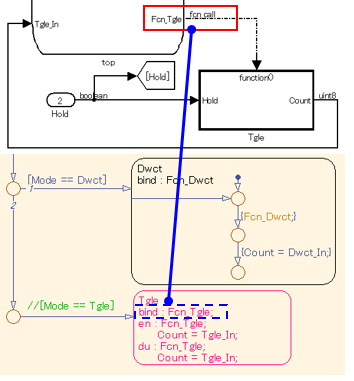### Function Types Available in Stateflow

The functions types used in Stateflow shall be dependent on the required processing.

For graphical functions, use:

• `If`, `elseif`, `else` logic

• Transfer functions

• Integrators

• Table look-ups

For MATLAB functions, use:

• Complex equations

• `If`, `elseif`, `else` logic